# Two Blocks Colide and Move Up A Ramp

Yet again a Physics problem for solving:

Two blocks are moving along a horizontal frictionless surface. The 4 kg block is moving to the right with a speed of 6 m/s and the 2 kg block is moving to the left with a speed of 3 m/s. The two blocks collide inelastically and then continue to move together along the surface and then up a 30° frictionless ramp. How high up the ramp does the combined object move?

Great!  To be honest this problem took me a little bit because I was making it quite a bit more complicated than was needed.  The easiest way to solve this is by breaking up the problem into 2 parts, solving the first with conservation of momentum and the second with simple linear kinematics.

So, let’s get down to business.  We have the following information that will be helpful to us.

Mass 1 = 4 kg
Velocity 1 = 6 m/s
Mass 2 =  2 kg
Combined Mass (they stick remember) = 6 kg
Velocity 2 = 3 m/s
Inclined Angle = 30 degrees
Gravitational Acceleration = 9.8 m/s^2

Now, we need to work out the first part.  We have to blocks moving in opposite directions that (by what the problem states) will stick together and continue moving at a certain velocity in a certain direction (by the problem we can assume toward the ramp).  So we use simple conservation of momentum as follows: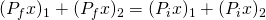Since they stick together, our result will only be one result of m * v.  It is also important to note that because the second mass is moving toward the first, the sign for the second set will be negative, without this, you’ll definitely get the wrong answer.We also need to remember that we have our final mass already by adding the blocks masses since they stuck together, 6 kg.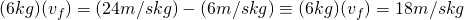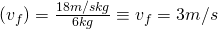Wonderful!  Now we know how fast the blocks are moving after they combine and, consequently, when they hit the bottom of the ramp.  Now, we know that the incline of the ramp is 30 degrees from horizontal (x).  Because of this, we need to modify our acceleration due to gravity as a result.  This is important to find because it will tell us exactly how fast the blocks are slowing down.  If we know that, then we can use one of our simple kinematics equations to solve for the distance (as you’ll see).

Now, saving a lot of vector breakdown, the formula needed to find the modified value of gravitational acceleration is: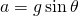Now we simply plug in our values: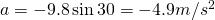So, we know that the velocity the blocks reach the ramp at is 3 m/s and the acceleration due to the 30 decree incline is -4.9 m/s^2 and that to find the distance up the ramp they go, the final velocity will be 0 because at that point the blocks will start going back down the ramp.  Using the formula below we can plug in our calculations to find the final distance.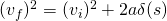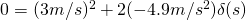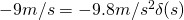### 1 Response

1.Pavel Holoborodko October 29, 2010 / 9:09 am

Hi!

I am author of WP-QuickLaTeX plugin for WordPress you are using for rendering equations on your blog.
Thank you for using it!

One tip. If you want equation to be centered on the page horizontally, you can use following syntax:

$$your LaTeX code$$

Best wishes.